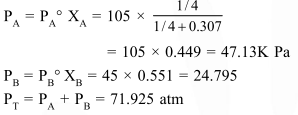# On mixing, heptane and octane form an ideal solution.

Question:

On mixing, heptane and octane form an ideal solution. At $373 \mathrm{~K}$, the vapour pressures of the two liquid components (heptane and octane) are $105 \mathrm{kPa}$ and $45 \mathrm{kPa}$ respectively. Vapour pressure of the solution obtained by mixing $25.0$ of heptane and $35 \mathrm{~g}$ of octane will be (molar mass of heptane $=100 \mathrm{~g} \mathrm{~mol}^{-1}$ and of octane $=114 \mathrm{~g} \mathrm{~mol}^{-1}$ ) :-

1.  144.5 kPa

2.  72.0 kPa

3. 36.1 kPa

4.  96.2 kPa

Correct Option: , 2

Solution: